##### Managerial Economics For Dummies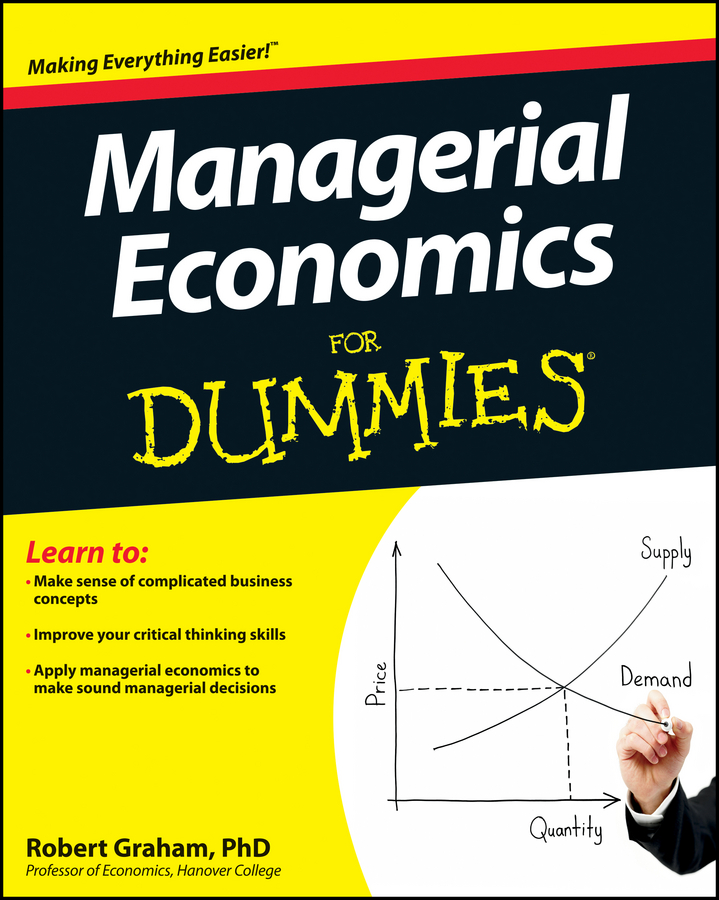A firm using breakeven analysis determines the smallest output level that leads to zero economic profit. Recall that zero economic profit doesn’t mean that the firm’s owners receive nothing — it means that the firm’s owners are receiving a normal rate of return. In other words, the firm’s owners are receiving exactly as much as they would in their next best alternative.

In breakeven pricing, your total revenue equals total cost — hence, zero profit. Because the focus is on the point where you earn zero profit, it’s unlikely that breakeven analysis maximizes your profit.

However, breakeven analysis is a useful managerial tool. Managers use breakeven analysis to determine how a price change affects profit. If you lower price, how many more units do you have to sell in order to achieve zero profit — or to break even? If your firm has a large fixed cost, breakeven analysis enables you to determine the quantity of output you must sell in order to avoid losses.

In either of these situations, as a manager, you can then determine whether or not sales of that amount are feasible.

Your company has total fixed cost of \$300,000, and its average variable cost (variable cost per unit of output) is \$2.00. In addition, you sell the good at a price of \$5.00 per unit. The following steps are used to determine the breakeven point:

1. Set total revenue equal to total cost.

Remember that total revenue equals price multiplied by the quantity sold, and total cost equals total fixed cost plus total variable cost.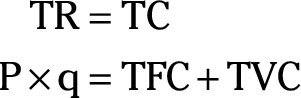2. Substitute AVC×q for TVC.

Recall that total variable cost equals average variable cost multiplied by the number of units produced q.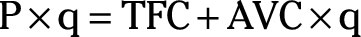3. Subtract AVC×q from both sides of the equation in Step 2 and simplify.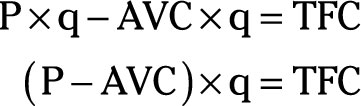4. Divide both sides of the equation by (P – AVC).

This step enables you to solve for the breakeven quantity, q.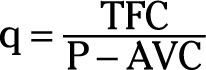5. Substitute the values for TFC, P, and AVC and solve for q.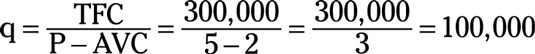Your breakeven quantity is 100,000 units.